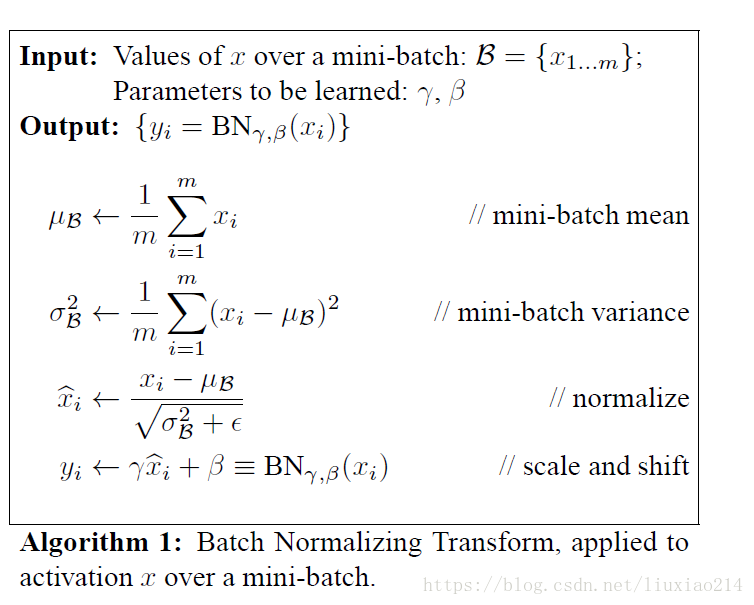• 也就是输入神经元和输出神经元随机一个数，然后除以输入神经元的个数开根号 因为全连接层波动较大，所以加在FC后面 这里Y,b是为了对信息的一个还原 转载于:...

w权值的初始化，之前最好的方法是也就是输入神经元和输出神经元中随机一个数，然后除以输入神经元的个数开根号因为全连接层波动较大，所以加在FC后面
这里Y,b是为了对信息的一个还原转载于:https://www.cnblogs.com/wuxiangli/p/6012618.html
展开全文• html 写的很好 ''' 本文讲解的是在CNN中的batch normalization ''' import torch import torch.nn as nn import copy class Net(nn.Module): def __init__(self, di...
https: // www.cnblogs.com / adong7639 / p / 9145911.
html
写的很好
'''
本文讲解的是在CNN中的batch normalization
'''
import torch
import torch.nn as nn
import copy

class Net(nn.Module):
def __init__(self, dim, pretrained):
super(Net, self).__init__()
self.bn = nn.BatchNorm2d(dim, 0)
if pretrained:
self.pretrained()

def forward(self, input):
return self.bn(input)

def pretrained(self):
nn.init.constant_(self.bn.weight, 1)
nn.init.constant_(self.bn.bias, 0)

def train():
dim = 3
model = Net(dim)

print(sum(p.numel() for p in model.parameters() if p.requires_grad))

for p in model.parameters():
'''
对于CNN特征图通道数为3的Batch normalization层而言，BN层的learnable parameter有6个，分别是gamma和beta
在训练过程中gamma和beta才是需要被更新的
6
Parameter containing:
Parameter containing:
'''

# model.eval()
feature_map = torch.randn((2, 3, 2, 2))

output1 = model(feature_map)
state_dict = model.state_dict()
for k, v in state_dict.items():
print(k, v)
'''
bn.weight tensor([0.2860, 0.5986, 0.0594])
bn.bias tensor([0., 0., 0.])
bn.running_mean tensor([-0.2098,  0.1876, -0.3045])
bn.running_var tensor([0.8099, 1.5140, 0.5880])
bn.num_batches_tracked tensor(1)
打印字典时，发现batch normalization层有5个参数
其中bn.weight 对应论文中的gamma   bn.bias对应论文中的beta
bn.running_mean则是对于当前batch size的数据所统计出来的平均值
bn.running_var是对于当前batch size的数据所统计出来的方差
'''
print('bn.running_mean', state_dict['bn.running_mean'])
print('bn.running_var', state_dict['bn.running_var'])
#
print(torch.mean(feature_map.permute(1, 0, 2, 3).contiguous().view(dim, -1), 1))
print(torch.var(feature_map.permute(1, 0, 2, 3).contiguous().view(dim, -1), 1))
'''
bn.running_mean tensor([-0.2098,  0.1876, -0.3045])
bn.running_var tensor([0.8099, 1.5140, 0.5880])

tensor([-0.2098,  0.1876, -0.3045])
tensor([0.8099, 1.5140, 0.5880])
当然这是在设定BN层的momentum=1时，即当前时刻的统计量（running_mean和running_var）完全由统计平均值决定
statistic_t_new=(1-momentum)*stastic_(t-1)+momentum)*stastic_(t)
momentum决定当前时刻的bn.running_mean和bn.running_var数值
（1）当momentum=1时，则数值完全由当前时刻计算出来的统计量决定
（2）由于模型上一次的统计量（由于这里不进行模型的参数更新和迭代训练，故而模型的初始值
bn.running_mean tensor([0., 0., 0.])
bn.running_var tensor([1., 1., 1.])）   可能不是0 0 0   1  1  1，而是随机初始化
故而如果将momentum设置为0，则模型会一直保持
bn.running_mean tensor([0., 0., 0.])
bn.running_var tensor([1., 1., 1.])
（3）当设置默认参数momentum=0.1时
bn.running_mean tensor([0.0233, 0.0166, 0.0469])
bn.running_var tensor([0.9961, 1.0899, 0.9974])
tensor([0.2329, 0.1663, 0.4691])    表示用tensor的方法计算出来的统计量
tensor([0.9615, 1.8986, 0.9738])
刚好bn.running_mean和bn.running_var是统计量的0.1倍

再次回顾计算BN的方式：
对于CNN的输入而言（即BN的输出时4-dimension），则
在batch，H,W 维度上进行normalization，也被称为spatial batch normalization
'''

if __name__ == '__main__':
'''
在BN层中，一般，bn.weight时随机初始化的，而bn.bias初始化为全0
假设现在已知输入特征图的数值，和对应batch normalization的参数，求BN输出的结果
momentum=0.1默认值    0.9*(t-1时刻的统计量)+0.1*(t时刻的统计量)
'''
dim = 3
momentum = 0.1
model = Net(dim, True)
input = torch.randn((2, 3, 2, 2))
output1 = model(input)

def bn_simple_train(input, model):
'''
:param input: 卷积神经网络特征图  shape [batch size,C,H,W]
:return:
'''
mean = torch.mean(input.permute(1, 0, 2, 3).contiguous().view(dim, -1), 1)  # shape [dim]
var = torch.var(input.permute(1, 0, 2, 3).contiguous().view(dim, -1), 1)  # shape [dim]

init_mean = torch.zeros((dim))
init_var = torch.ones((dim))

run_mean = (1 - momentum) * init_mean + momentum * mean  # 滑动平均的方式计算新的均值，训练时计算，为测试数据做准备
run_var = (1 - momentum) * init_var + momentum * var  # 滑动平均的方式计算新的方差，训练时计算，为测试数据做准备

run_std = torch.sqrt(run_var + 1e-5)

run_mean_exp = run_mean.view(1, input.shape, 1, 1).expand(input.shape)
run_std_exp = run_std.view(1, input.shape, 1, 1).expand(input.shape)
'''
这里的tensor复制问题也让我想了很久

tensor1=torch.tensor([1,2,3])
需要得到一个2*3*2*2的tensor2，然后需要满足
tensor2[:,0,:,:]=1
tensor2[:,1,:,:]=2
tensor2[:,2,:,:]=3
这个，除了for循环，内部函数也可以实现

先unsqueeze 到 ，(1， 2， 1， 1)  再 expand(2，3，2，2)
expand, 只能再指定维度进行复制， 不能增加维度， 所以你要先unsqueeze到4个维度
expand的时候会找channel相同的维度，这些维度不变，其他维度复制

'''

# run_mean_exp=torch.zeros((2,3,2,2))
# for i in range(3):
#     run_mean_exp[:,i,:,:]=run_mean[i]

# run_std_exp = torch.zeros((2, 3, 2, 2))
# for i in range(3):
#     run_std_exp[:, i, :, :] = run_std[i]

output2 = input - run_mean_exp
output2 = output2 / run_std_exp

init_weights = model.state_dict().items()['bn.weights']  # gamma
init_bias = model.state_dict().items()['bn.bias']  # beta
init_weights_exp = init_weights.view(1, input.shape, 1, 1).expand(input.shape)
init_bias_exp = init_bias.view(1, input.shape, 1, 1).expand(input.shape)
'''
在训练过程中会一直更新（反向传播时）的可学习参数
'''
# init_weights_exp=torch.zeros((2, 3, 2, 2))
# for i in range(3):
#     init_weights_exp[:, i, :, :] = init_weights[i]
#
# init_bias_exp = torch.zeros((2, 3, 2, 2))
# for i in range(3):
#     init_bias_exp[:, i, :, :] = init_bias[i]
output2 = output2 * init_weights_exp
output2 = output2 + init_bias_exp

return output2

def bn_for_test(input, model):
'''
测试过程中，BN层的running mean和running var都是固定值，不再时新的验证数据的统计量，在model.eval()模式下这两个参数会被固定住
而gamma和beta也不发生改变
:param input:
:param model:
:return:
'''
state_dict = model.state_dict()
init_weights = state_dict.items()['bn.weight']
init_bias = state_dict.items()['bn.bias']
running_mean = state_dict.items()(['bn.running_mean']
running_var = state_dict.tems()['bn.running_var']

mean = running_mean.view(1, input.shape, 1, 1).expand(input.shape)
var = running_var.view(1, input.shape, 1, 1).expand(input.shape)
weights = init_weights.view(1, input.shape, 1, 1).expand(input.shape)
bias = init_bias.view(1, input.shape, 1, 1).expand(input.shape)

output = (input - mean) / torch.sqrt(var + 1e-5)
output = output * weights + bias
return output
展开全文• CNN中，Batch就是训练网络所设定的图片数量batch_size。 Normalization过程： 输入：输入数据x1…xm（这些数据是准备进入激活函数的数据） 计算过程可以看到, 1.求数据均值； 2.求数据方差； 3.数据进行标准化...
什么是BN
Batch Normalization（数据批量标准化）是由google提出的一种训练优化方法。 先说Batch是怎么确定的。在CNN中，Batch就是训练网络所设定的图片数量batch_size。 Normalization过程：输入：输入数据x1…xm（这些数据是准备进入激活函数的数据） 计算过程中可以看到, 1.求数据均值； 2.求数据方差； 3.数据进行标准化（个人认为称作正态化也可以） 4.训练参数γ，β 5.输出y通过γ与β的线性变换得到新的值
在正向传播的时候，通过可学习的γ与β参数求出新的分布值；在反向传播的时候，通过链式求导方式，求出γ与β以及相关权值。 链式求导法则：BN的作用
（1）防止梯度消失与梯度爆炸
梯度消失： 以sigmoid函数为例子，sigmoid函数使得输出在[0,1]之间。事实上x到了一定大小，经过sigmoid函数的输出范围就很小了，参考下图。如果输入很大，其对应的斜率就很小，我们知道，其斜率（梯度）在反向传播中是权值学习速率。所以就会出现如下的问题。在深度网络中，如果网络的激活输出很大，其梯度就很小，学习速率就很慢。假设每层学习梯度都小于最大值0.25，网络有n层，因为链式求导的原因，第一层的梯度小于0.25的n次方，所以学习速率就慢，对于最后一层只需对自身求导1次，梯度就大，学习速率就快。 这会造成的影响是在一个很大的深度网络中，浅层基本不学习，权值变化小，后面几层一直在学习，结果就是，后面几层基本可以表示整个网络，失去了深度的意义。 梯度爆炸： 根据链式求导法， 第一层偏移量的梯度=激活层斜率1x权值1x激活层斜率2x…激活层斜率(n-1)x权值(n-1)x激活层斜率n 假如激活层斜率均为最大值0.25，所有层的权值为100，这样梯度就会指数增加。
（2）允许更大的学习率，大幅提高训练速度
你可以选择比较大的初始学习率，让你的训练速度飙涨。以前还需要慢慢调整学习率，甚至在网络训练到一半的时候，还需要想着学习率进一步调小的比例选择多少比较合适，现在我们可以采用初始很大的学习率，然后学习率的衰减速度也很大，因为这个算法收敛很快。当然这个算法即使你选择了较小的学习率，也比以前的收敛速度快，因为它具有快速训练收敛的特性。
（3）改善正则化策略：作为正则化的一种形式，轻微减少了对dropout的需求
你再也不用去理会过拟合中drop out、L2正则项参数的选择问题，采用BN算法后，你可以移除这两项了参数，或者可以选择更小的L2正则约束参数了，因为BN具有提高网络泛化能力的特性。
（4）减少对初始化的强烈依赖
（5）不需要使用局部响应归一化层了，因为BN本身就是一个归一化网络层
（6）可以把训练数据彻底打乱（防止每批训练的时候，某一个样本都经常被挑选到，文献说这个可以提高1%的精度）
在CNN中使用BN
BN层同卷积层、池化层一样，都是独立的一层，并且一般用在卷积层和线性层之后，因为非线性单元的输出分布形状会在训练过程中变化，归一化无法消除他的方差偏移，相反的，全连接和卷积层的输出一般是一个对称,非稀疏的一个分布，更加类似高斯分布，对他们进行归一化会产生更加稳定的分布。以上图卷积为例，蓝色层为输入，绿色为卷积后的特征图。特征图里的值，作为BN的输入，也就是这4个数值通过BN计算并保存γ与β，通过γ与β使得输出与输入不变。假设输入的batch size为m，那就有m4个数值，计算这m4个数据的γ与β并保存。正向传播过程如上述，对于反向传播就是根据求得的γ与β计算梯度。
需要注意两点：
1.网络训练中以batch size为最小单位不断迭代，很显然，新的batch size进入网络，机会有新的γ与β，因此，在BN层中，有总图片数/batch size组γ与β被保存下来。 2.图像卷积的过程中，通常是使用多个卷积核，得到多张特征图，对于多个的卷积核需要保存多个的γ与β。
结合论文中给出的使用过程进行解释：输入：待进入激活函数的变量 输出： 1.对于K维的输入，假设每一维包含m个变量，所以需要K个循环。每个循环中按照上面所介绍的方法计算γ与β。这里的K维，在卷积网络中可以看作是卷积核个数，如网络中第n层有64个卷积核，就需要计算64次。 需要注意，在正向传播时，会使用γ与β使得BN层输出与输入一样 （训练集时）。 2.在反向传播时利用γ与β求得梯度从而改变训练权值（变量）。 3.通过不断迭代直到训练结束，求得关于不同层的γ与β。如网络有n个BN层，每层根据batch_size决定有多少个变量，设定为m，这里的mini-batcherB指的是特征图大小batch_size，即m=特征图大小*batch_size，因此，对于batch_size为1时，这里的m就是每层特征图的大小。 4.不断遍历训练集中的图片，取出每个batch_size中的γ与β，最后统计每层BN的γ与β各自的和除以图片数量得到平均直，并对其做无偏估计直作为每一层的E[x]与Var[x]。 5.在预测的正向传播时，对测试数据求取γ与β，并使用该层的E[x]与Var[x]，通过图中11:所表示的公式计算BN层输出。 注意，在预测时，BN层的输出已经被改变（测试集时），所以BN层在预测的作用体现在此处。
BN的pytorch代码实现
Pytorch中的BN操作为：
nn.BatchNorm2d(self, num_features, eps=1e-5, momentum=0.1, affine=True, track_running_stats=True)

num_features：输入数据的通道数，归一化时需要的均值和方差是在每个通道中计算的
eps：用来防止归一化时除以0

momentum：滑动平均的参数，用来计算running_mean和running_var

affine：是否进行仿射变换，即缩放操作

track_running_stats：是否记录训练阶段的均值和方差，即running_mean和running_var

BN层的状态包含五个参数:
weight：缩放操作的γ。
bias：缩放操作的β。
running_mean：训练阶段统计的均值，测试阶段会用到。
running_var：训练阶段统计的方差，测试阶段会用到。
num_batches_tracked：训练阶段的batch的数目，如果没有指定momentum，则用它来计算running_mean和running_var。一般momentum默认值为0.1，所以这个属性暂时没用。
weight和bias这两个参数需要训练，而running_mean、running_val和num_batches_tracked不需要训练，它们只是训练阶段的统计值。
  #示例
bn = nn.BatchNorm2d(3)
x = torch.randn(4, 3, 2, 2)
y = bn(x) #前向传播一次
a = (x[0, 0, :, :] + x[1, 0, :, :] + x[2, 0, :, :] + x[3, 0, :, :]).sum() / 16
b = (x[0, 1, :, :] + x[1, 1, :, :] + x[2, 1, :, :] + x[3, 1, :, :]).sum() / 16
c = (x[0, 2, :, :] + x[1, 2, :, :] + x[2, 2, :, :] + x[3, 2, :, :]).sum() / 16
print('The mean value of the first channel is %f' % a.data)
print('The mean value of the first channel is %f' % b.data)
print('The mean value of the first channel is %f' % c.data)
print('The output mean value of the BN layer is %f, %f, %f' % (bn.running_mean.data, bn.running_mean.data, bn.running_mean.data))

展开全文• 对轴承的时频图进行特征提取与融合，构建轴承健康指示量
• （1）BN计算就是把每个通道的NHW单独拿出来进行计算：就是在每一个批次的第一个通道进行计算，因此，nn.BatchNorm()填的是通道数，就是将一批次的每个通道都计算一次 （2）针对每个channel我们兜兜一组gamma和...
Noramal详解Vlog1
BatchNormal

y

=

x

−

E

[

x

]

V

a

r

[

x

]

+

C

∗

γ

+

β

y=\frac{x-E[x]}{\sqrt{Var[x]+C}}*γ+βBatch Normalization （1）BN计算就是把每个通道的NHW单独拿出来进行计算：就是在每一个批次中的第一个通道进行计算，因此，nn.BatchNorm()中填的是通道数，就是将一批次中的每个通道都计算一次 （2）针对每个channel我们兜兜一组gamma和beta，所以可以学习的参数为（2*通道数） （3）当batch size比较小时，BN的表现效果也越不好，因为计算过程中所得到的均值和方差不能代表全局(1)沿着通道计算每个batch的均值u (2)沿着通道计算每个batch的方差σ^2 (3)对x做归一化，x’=(x-u)/开根号(σ^2+ε) (4)加入缩放和平移变量γ和β ,归一化后的值，y=γx’+β

LayerNormalLayer Normalization （1）LN的计算就是把每个CHW单独拿出来记性计算，就是将每一个图片进行的3个通道进行计算，不受batch size的影响 （2）常常用在RNN网络中，但如果输入的特征区别很大，那么就不建议使用它做归一化处理

InstanceNormalInstance Noramlization （1）IN的计算就是把每个HW单独拿出来归一化处理，不受通道和batch size的影响 （2）常用在风格化迁移，但如果特征图可以用到通道之间的相关性，那么久不建议使用它做归一化处理。

GroupNormalGroup Noramlization （1）GN的计算先把通道C分成Group组，然后把每个gHW单独拿出来做归一化处理，然后把Group组归一化之后的数据合并成CHW （2）GN介于LN和IN之间，当然也可以说LN和IN就是GN的特例，比如Group的大小为1或者C

Switchable NormalizaionSwitchable Normalizaion （1）将BN、IN、LN结合，赋予权重，让网络学习去归一化层应该使用什么方法 （2）集万千宠爱与一身，但是训练复杂总结展开全文• 看到BN后，很明显能够看到这等于往传统的神经网络增加了一个BN层，而且位置处于神经元非线性变换前，基本大多数网络结构都能很自然地融合进去，于是很自然的想法就是：如果用在CNN或者RNN效果会如何？是否也会增加...RNN
• 前言 Batch Normalization是由google提出的一种训练优化方法。参考论文：Batch ...个人觉得BN层的作用是加快网络学习速率，论文提及其它的优点都是这个优点的副产品。  网上对BN解释详细的不多，大多从...
• 在看 ladder network(https://arxiv.org/pdf/1507.02672v2.pdf) 时初次遇到batch normalization（BN）. 文中说BN能加速收敛等好处，但是并不理解，然后就在网上搜了些...看了知乎上关于深度学习 Batch Normaliza...
• 时间: 2020/06/18 ...魔改的cnn的推理时，将bn折叠，即在训练的变量上乘以一个系数从而将bn层在推理时舍去， from __future__ import absolute_import from __future__ import division from __future__ import pr.
• BatchNorm2d原理、作用及其pytorchBatchNorm2d函数的参数讲解： https://my.oschina.net/u/4274857/blog/4512852 自己绘制的图如下：html html5
• 1 致谢 感谢网友wzy_zju提供的资料...我之前一直对BN的作用还不是很明白，所以今天再来复习一下~ 3 Batch Normalization的算法思路 这里我们可以参考一下原始论文的算法图： 可以看到，这里γ\gammaγ和β\betaβ ...
• BN(BatchNormalization)层的作用： 1，加速收敛； ...1，网络训练以batch_size为最小单位不断迭代，新的batch_size进入网络，就会产生新的γ与β，在BN，有总图片/batch_size组γ与β被保...
• 作者：陈永志 ... 来源：知乎 著作权归作者所有。商业转载请联系作者获得授权，非商业转载请注明出处。 ...一、weight decay（权值衰减）的使用既不是为了提高你所说的收敛精确度也...在损失函数，weight decay是放在正正则 batch momentum神经网络...